# Selina Solutions Concise Mathematics Class 6 Chapter 23 Fundamental Concepts Exercise 23(B)

Selina Solutions Concise Mathematics Class 6 Chapter 23 Fundamental Concepts Exercise 23(B) explains the basic concepts, based on perpendicular lines. The solutions can be used by the students in practicing the exercise problems, without any time limits. Experts prepare solutions, as per the current ICSE syllabus for Class 6. Students, who follow these solutions, undoubtedly boost their confidence in solving the problems without any difficulty. For in-depth knowledge about the concepts covered, students can use Selina Solutions Concise Mathematics Class 6 Chapter 23 Fundamental Concepts Exercise 23(B) PDF, from the links given below.

## Selina Solutions Concise Mathematics Class 6 Chapter 23 Fundamental Concepts Exercise 23(B) Download PDF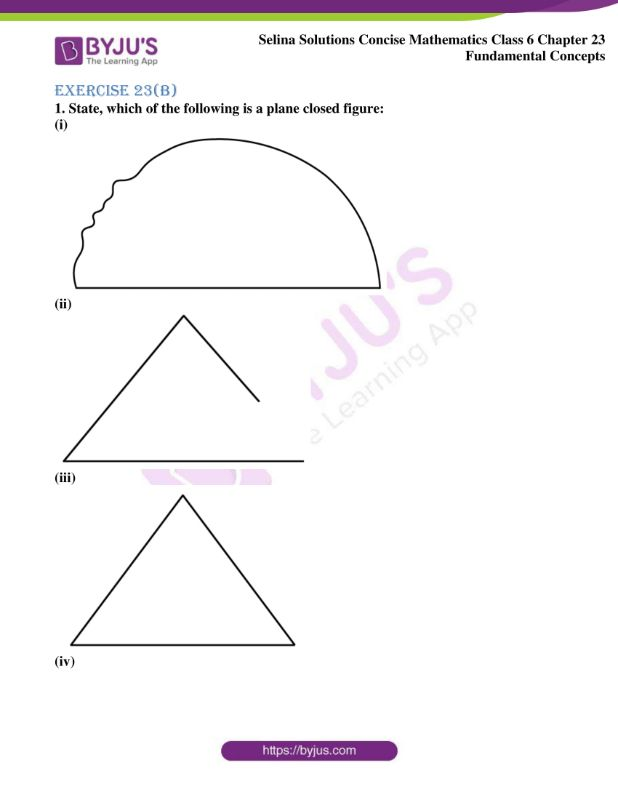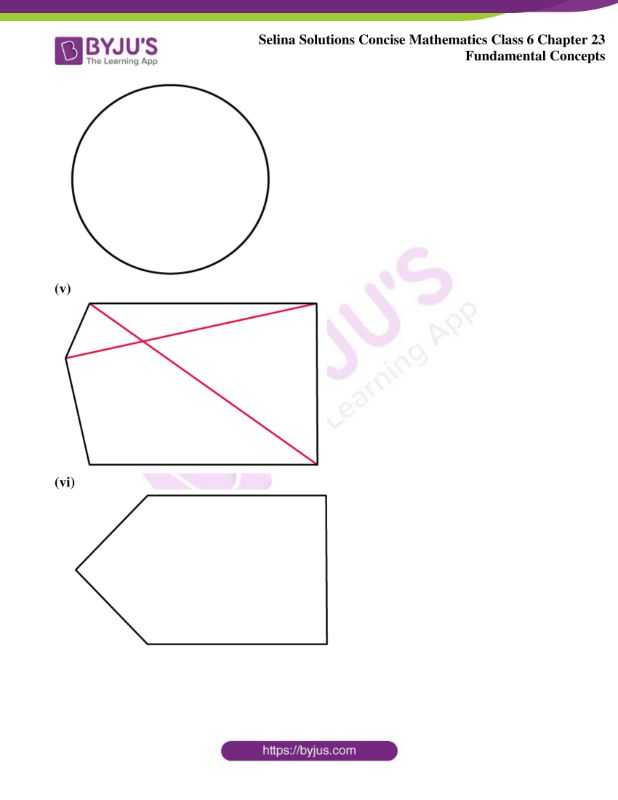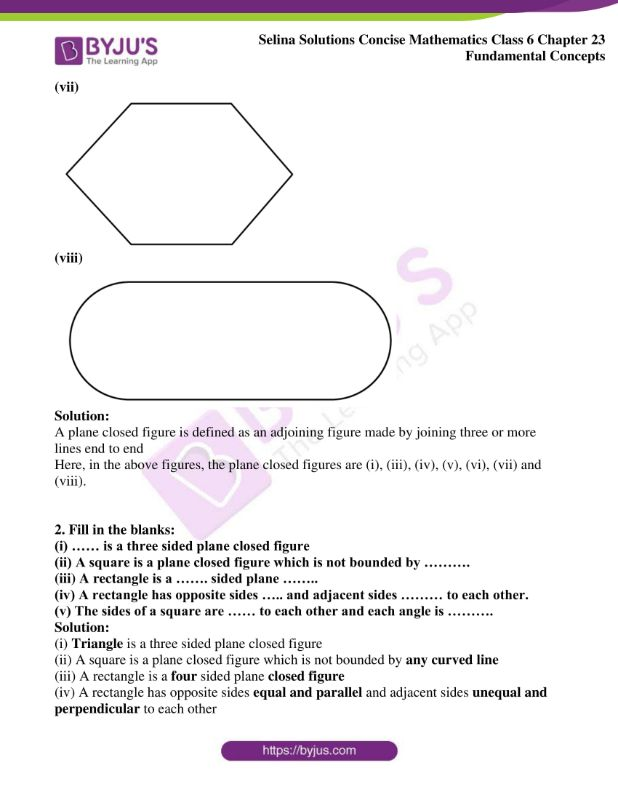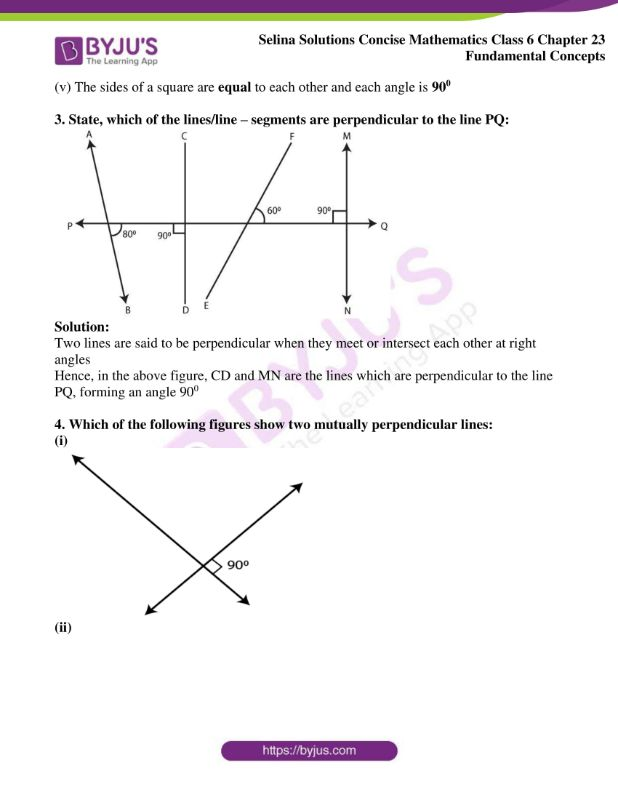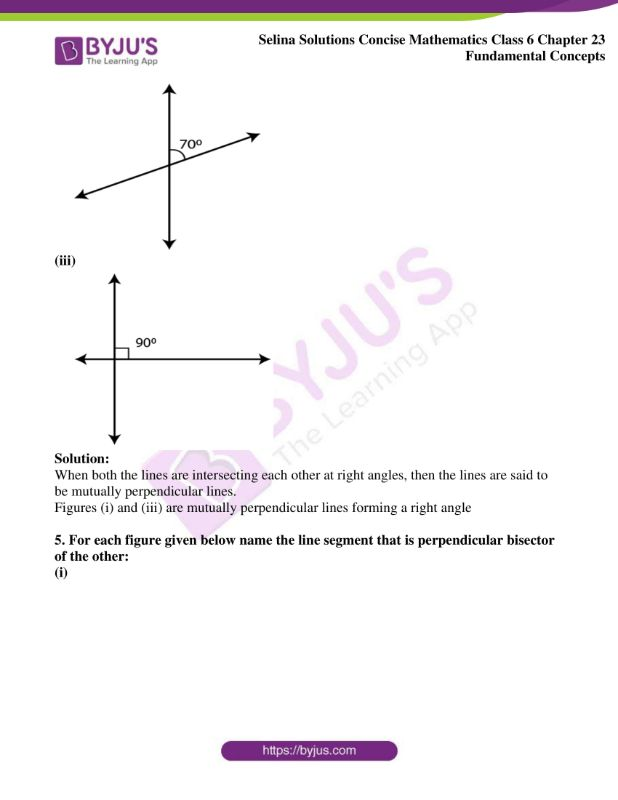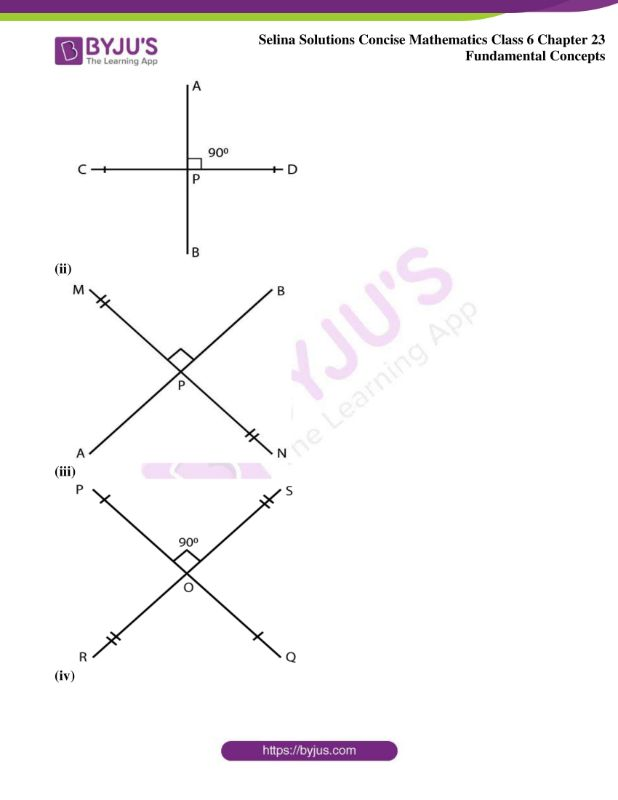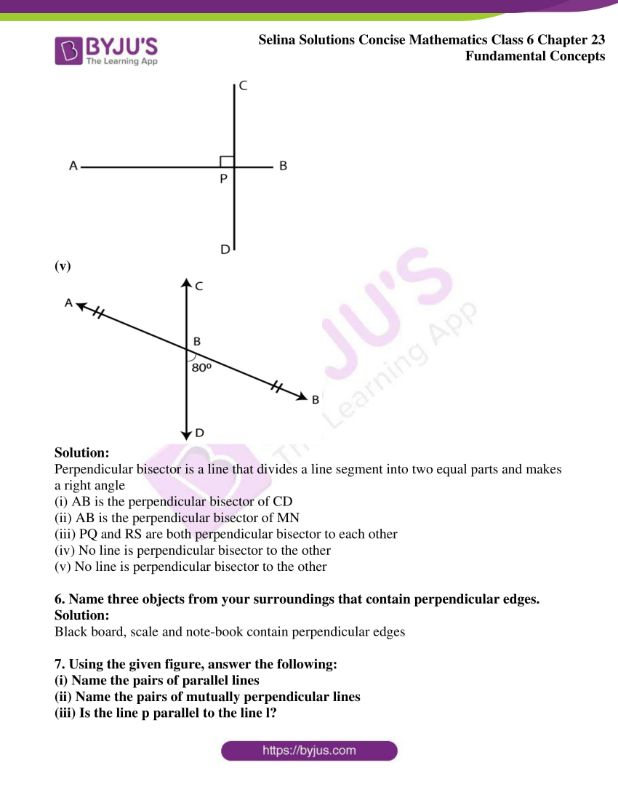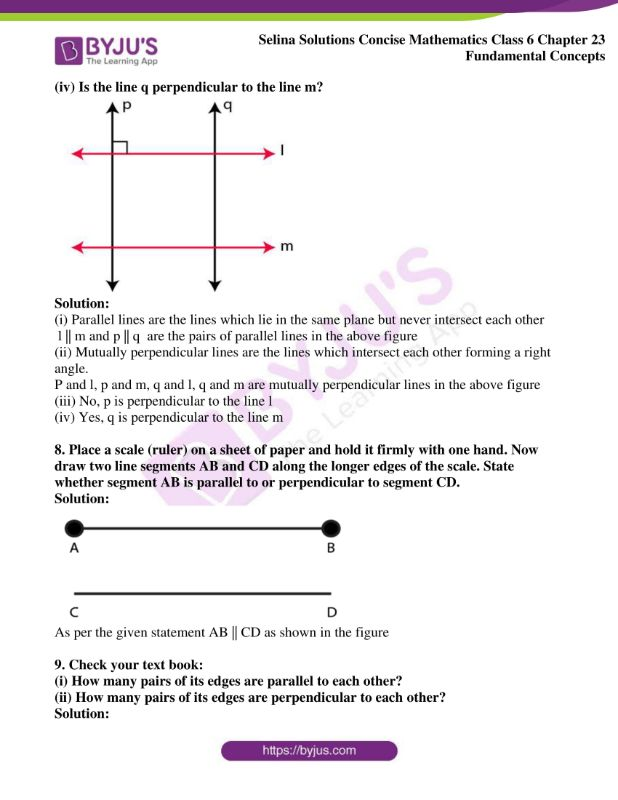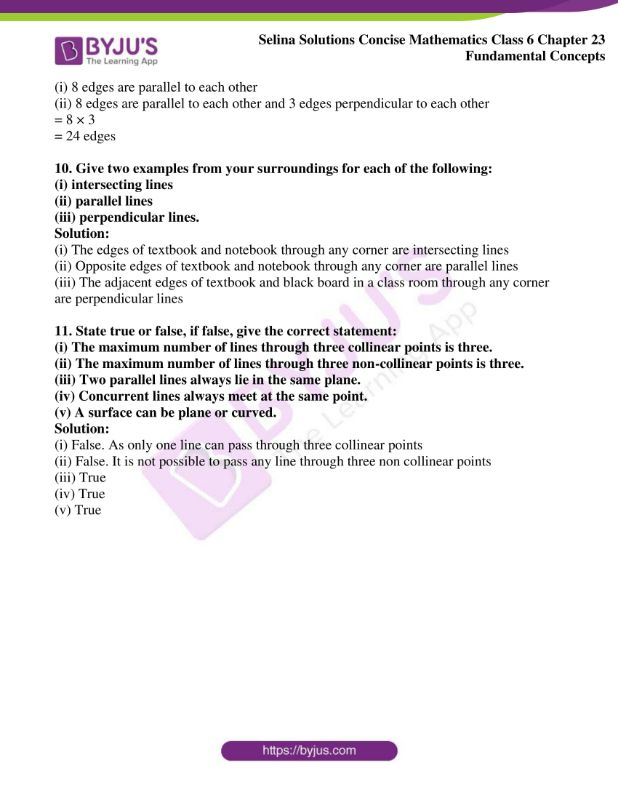### Access another exercise of Selina Solutions Concise Mathematics Class 6 Chapter 23 Fundamental Concepts

Exercise 23(A) Solutions

### Access Selina Solutions Concise Mathematics Class 6 Chapter 23 Fundamental Concepts Exercise 23(B)

Exercise 23(B)

1. State, which of the following is a plane closed figure:

(i)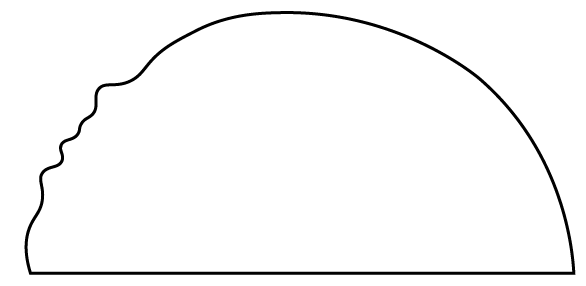(ii)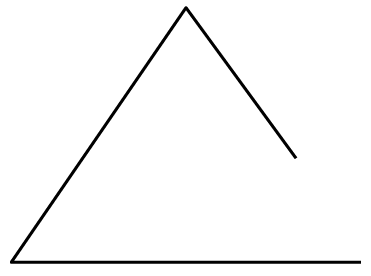(iii)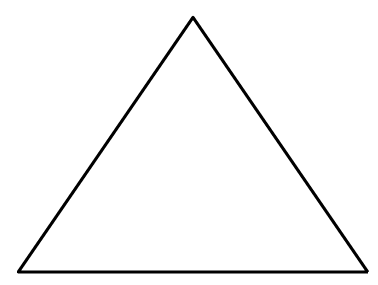(iv)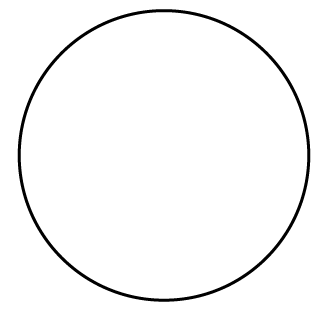(v)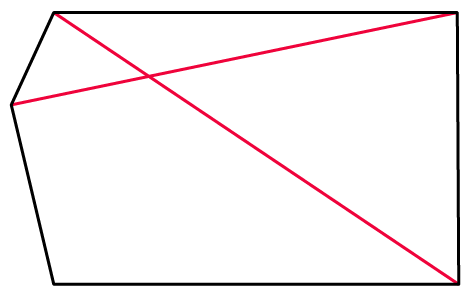(vi)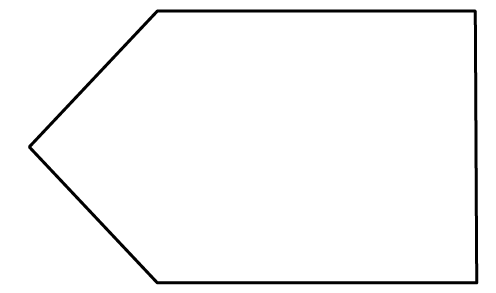(vii)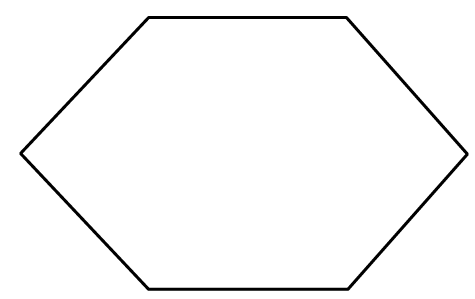(viii)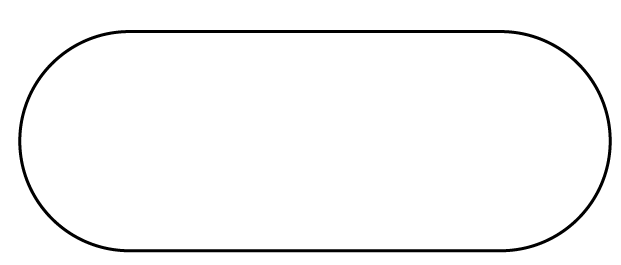Solution:

A plane closed figure is defined as an adjoining figure made by joining three or more lines end to end

Here, in the above figures, the plane closed figures are (i), (iii), (iv), (v), (vi), (vii) and (viii).

2. Fill in the blanks:

(i) …… is a three sided plane closed figure

(ii) A square is a plane closed figure which is not bounded by ……….

(iii) A rectangle is a ……. sided plane ……..

(iv) A rectangle has opposite sides ….. and adjacent sides ……… to each other.

(v) The sides of a square are …… to each other and each angle is ……….

Solution:

(i) Triangle is a three sided plane closed figure

(ii) A square is a plane closed figure which is not bounded by any curved line

(iii) A rectangle is a four sided plane closed figure

(iv) A rectangle has opposite sides equal and parallel and adjacent sides unequal and perpendicular to each other

(v) The sides of a square are equal to each other and each angle is 900

3. State, which of the lines/line – segments are perpendicular to the line PQ: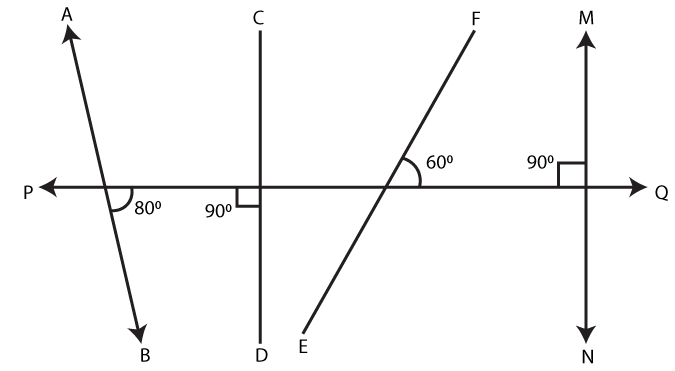Solution:

Two lines are said to be perpendicular when they meet or intersect each other at right angles

Hence, in the above figure, CD and MN are the lines which are perpendicular to the line PQ, forming an angle 900

4. Which of the following figures show two mutually perpendicular lines:

(i)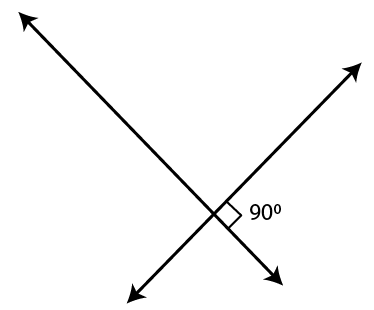(ii)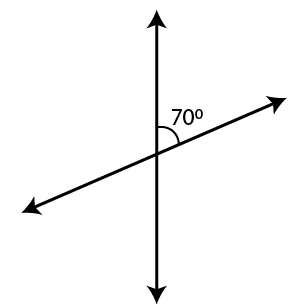(iii)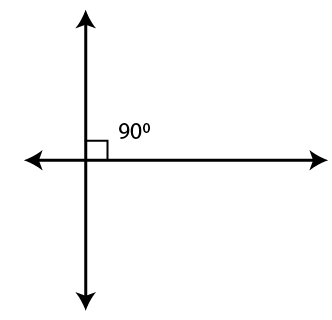Solution:

When both the lines are intersecting each other at right angles, then the lines are said to be mutually perpendicular lines.

Figures (i) and (iii) are mutually perpendicular lines forming a right angle

5. For each figure given below name the line segment that is perpendicular bisector of the other:

(i)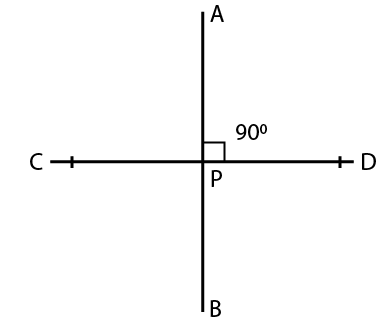(ii)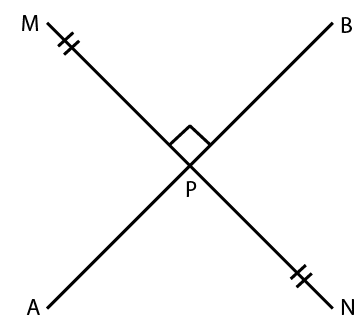(iii)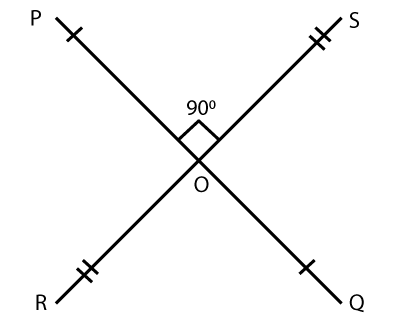(iv)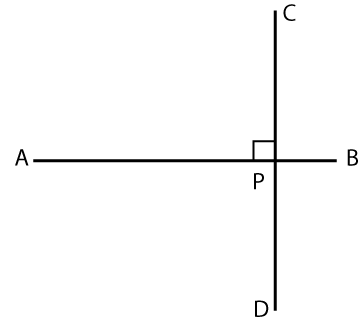(v)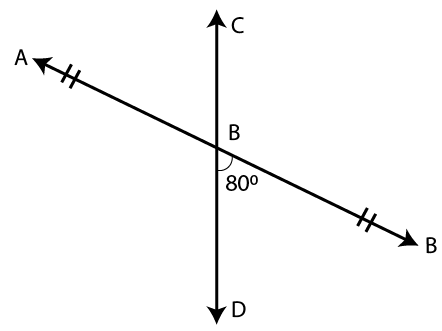Solution:

Perpendicular bisector is a line that divides a line segment into two equal parts and makes a right angle

(i) AB is the perpendicular bisector of CD

(ii) AB is the perpendicular bisector of MN

(iii) PQ and RS are both perpendicular bisector to each other

(iv) No line is perpendicular bisector to the other

(v) No line is perpendicular bisector to the other

6. Name three objects from your surroundings that contain perpendicular edges.

Solution:

Black board, scale and note-book contain perpendicular edges

7. Using the given figure, answer the following:

(i) Name the pairs of parallel lines

(ii) Name the pairs of mutually perpendicular lines

(iii) Is the line p parallel to the line l?

(iv) Is the line q perpendicular to the line m?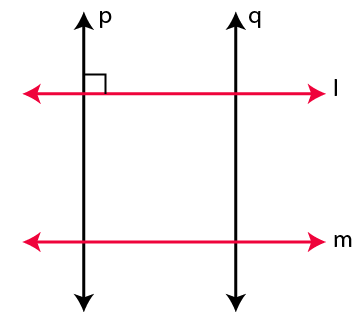Solution:

(i) Parallel lines are the lines which lie in the same plane but never intersect each other

l || m and p || q are the pairs of parallel lines in the above figure

(ii) Mutually perpendicular lines are the lines which intersect each other forming a right angle.

P and l, p and m, q and l, q and m are mutually perpendicular lines in the above figure

(iii) No, p is perpendicular to the line l

(iv) Yes, q is perpendicular to the line m

8. Place a scale (ruler) on a sheet of paper and hold it firmly with one hand. Now draw two line segments AB and CD along the longer edges of the scale. State whether segment AB is parallel to or perpendicular to segment CD.

Solution: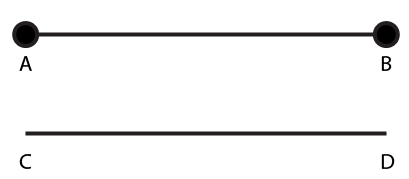As per the given statement AB || CD as shown in the figure

(i) How many pairs of its edges are parallel to each other?

(ii) How many pairs of its edges are perpendicular to each other?

Solution:

(i) 8 edges are parallel to each other

(ii) 8 edges are parallel to each other and 3 edges perpendicular to each other

= 8 × 3

= 24 edges

10. Give two examples from your surroundings for each of the following:

(i) intersecting lines

(ii) parallel lines

(iii) perpendicular lines.

Solution:

(i) The edges of textbook and notebook through any corner are intersecting lines

(ii) Opposite edges of textbook and notebook through any corner are parallel lines

(iii) The adjacent edges of textbook and black board in a class room through any corner are perpendicular lines

11. State true or false, if false, give the correct statement:

(i) The maximum number of lines through three collinear points is three.

(ii) The maximum number of lines through three non-collinear points is three.

(iii) Two parallel lines always lie in the same plane.

(iv) Concurrent lines always meet at the same point.

(v) A surface can be plane or curved.

Solution:

(i) False. As only one line can pass through three collinear points

(ii) False. It is not possible to pass any line through three non collinear points

(iii) True

(iv) True

(v) True